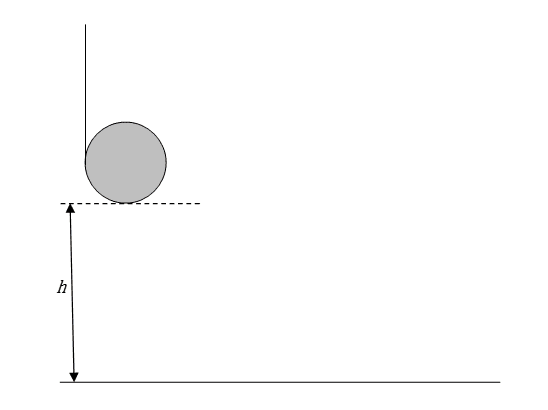# Falling Yo-Yo (angular momentum/energy, torque)

• kayem

## Homework Statement

Today I had a physics midterm and one question that was on it is really bugging me and my friend. We'd really appreciate it if one of the experts here could give us their spin on it (I know...bad pun ;)).

The question is:

A yo-yo (a solid cylinder with a string wrapped around it) is dropped from a height, h=1.0m . How long does it take to hit the ground? Think carefully about all the forces and torques acting on it.## The Attempt at a Solution

So we know that
$\tau=rF=I\alpha$

The force is supplied by gravity so $$F=mg$$ and the moment of inertia of a cylinder is $\frac{1}{2}mr^{2}$ and $a = \frac{\alpha}{r}$ so:

$mrg=\frac{1}{2}mr^{2}\frac{a}{r}$

Solving for translational (linear) acceleration, I get $a=2g$ which in my mind is incorrect since there's no way that attaching a string to a cylinder could make it accelerate twice as fast as freefall. I didn't know what else to do, so I kept on with that equation and used kinematics to find time of fall:

$\Delta s=\frac{1}{2}at^{2}$, $a=2g$
$t = \sqrt{2\Delta s a}$

Solving with $\Delta s=h=1.0$m and $a=2g$ I get:

$t= \sqrt{4g} = \sqrt{(4)(9.8)} = 6.3s$

Does this make any sense? Are we doing it right?

I also tried with energy:

$U_{G}=K_{translational}+K_{rotational}$
$mgh=\frac{1}{2}mv^{2} + \frac{1}{2}I\omega^{2}$

but I ended up with only a final velocity (as expected) and not enough information to solve for time.